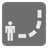Follow Instructions
Elementary+
English RU

You’ve received a letter from a friend whom you haven't seen or heard from for a while. In this letter he gives you instructions on how to find a hidden treasure.

In this mission you should follow a given list of instructions which will get you to a certain point on the map. A list of instructions is a string, each letter of this string points you in the direction of your next step.

The letter "f" - tells you to move forward, "b" - backward, "l" - left, and "r" - right.

It means that if the list of your instructions is "fflff" then you should move forward two times, make one step to the left and then again move two times forward.

Now, let's imagine that you are in the position (0,0). If you move forward your position will change to (0, 1). If you move again it will be (0, 2). If your next step is to the left then your position will become (-1, 2). After the next two steps forward your coordinates will be (-1, 4)

Your goal is to find your final coordinates. Like in our case they are (-1, 4)

Input: A string.

Output: An array of two ints

Example:

```follow('fflff') == [-1, 4]
follow('ffrff') == [1, 4]
follow('fblr') == [0, 0]
```

How it is used: In games with a map

Precondition: only chars f,b,l and r are allowed